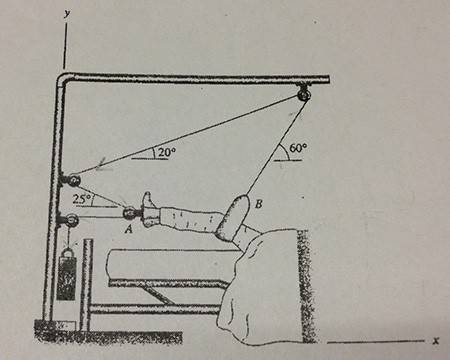# Pulley Traction in Equilibrium

• Missnomer
MENDMENT: In summary, the sum of downward forces exerted at points A and B is 41lbs by the leg. After considering the tension and vertical forces, it is concluded that there is a net horizontal force on the leg that the leg must resist. This can be attributed to the friction between the patient and the bed.

## Homework Statement

Sum of downward forces exerted at A and B is 41lbs by the leg, what is the weight attached?None, really...

## The Attempt at a Solution

Tension (T) throughout the system must be constant and equal to the weight (W).
I first assumed that the leg was entirely pulled up by B since I thought the force passing A
is going down (wrong since this is in equilibrium) and claimed W = 41sin60, but it's not that simple.

Tension pulls both ways, so there is an upward force at A as well as B.
Since
$ƩF_y = 0$
$Tsin25 + Tsin60 - 41lb = 0$
$T = W = 41 / (sin25 + sin60) = 31.0lb$

But using this value of T in checking horizontal forces, they don't balance out.
What am I missing here, or are my calculations correct and there just is a net horizontal force
on the leg that the leg has to resist?

Missnomer said:

## Homework Statement

Sum of downward forces exerted at A and B is 41lbs by the leg, what is the weight attached?None, really...

## The Attempt at a Solution

Tension (T) throughout the system must be constant and equal to the weight (W).
I first assumed that the leg was entirely pulled up by B since I thought the force passing A
is going down (wrong since this is in equilibrium) and claimed W = 41sin60, but it's not that simple.

Tension pulls both ways, so there is an upward force at A as well as B.
Since
$ƩF_y = 0$
$Tsin25 + Tsin60 - 41lb = 0$
$T = W = 41 / (sin25 + sin60) = 31.0lb$

But using this value of T in checking horizontal forces, they don't balance out.
What am I missing here, or are my calculations correct and there just is a net horizontal force
on the leg that the leg has to resist?